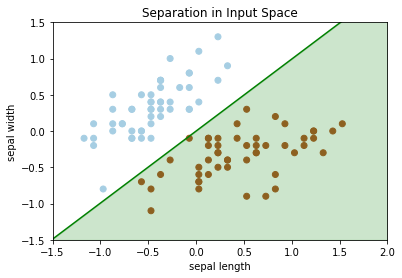# CSC321 Tutorial 3: Linear Classification¶

In this tutorial, we'll go through an example of linear classification. In addition, there should be some time towards the end of the tutorial to talk about project 1.

• set up the binary linear classification problem using numpy
• use the Iris flower dataset as a running example for classification
• explore the geometry of the problem
In :
import matplotlib
import numpy as np
import matplotlib.pyplot as plt
%matplotlib inline


## The Iris Flower Dataset¶

The Iris flower dataset is another one of the "toy datasets" available in sklearn.

We will only work with the first 2 flower classes (Setosa and Versicolour), and with just the first two features: length and width of the sepal

If you don't know what the sepal is, see this diagram: https://www.math.umd.edu/~petersd/666/html/iris_with_labels.jpg

We can import and display the dataset description like this:

In :
from sklearn.datasets import load_iris
print(iris['DESCR'])

.. _iris_dataset:

Iris plants dataset
--------------------

**Data Set Characteristics:**

:Number of Instances: 150 (50 in each of three classes)
:Number of Attributes: 4 numeric, predictive attributes and the class
:Attribute Information:
- sepal length in cm
- sepal width in cm
- petal length in cm
- petal width in cm
- class:
- Iris-Setosa
- Iris-Versicolour
- Iris-Virginica

:Summary Statistics:

============== ==== ==== ======= ===== ====================
Min  Max   Mean    SD   Class Correlation
============== ==== ==== ======= ===== ====================
sepal length:   4.3  7.9   5.84   0.83    0.7826
sepal width:    2.0  4.4   3.05   0.43   -0.4194
petal length:   1.0  6.9   3.76   1.76    0.9490  (high!)
petal width:    0.1  2.5   1.20   0.76    0.9565  (high!)
============== ==== ==== ======= ===== ====================

:Missing Attribute Values: None
:Class Distribution: 33.3% for each of 3 classes.
:Creator: R.A. Fisher
:Donor: Michael Marshall (MARSHALL%PLU@io.arc.nasa.gov)
:Date: July, 1988

The famous Iris database, first used by Sir R.A. Fisher. The dataset is taken
from Fisher's paper. Note that it's the same as in R, but not as in the UCI
Machine Learning Repository, which has two wrong data points.

This is perhaps the best known database to be found in the
pattern recognition literature.  Fisher's paper is a classic in the field and
is referenced frequently to this day.  (See Duda & Hart, for example.)  The
data set contains 3 classes of 50 instances each, where each class refers to a
type of iris plant.  One class is linearly separable from the other 2; the
latter are NOT linearly separable from each other.

.. topic:: References

- Fisher, R.A. "The use of multiple measurements in taxonomic problems"
Annual Eugenics, 7, Part II, 179-188 (1936); also in "Contributions to
Mathematical Statistics" (John Wiley, NY, 1950).
- Duda, R.O., & Hart, P.E. (1973) Pattern Classification and Scene Analysis.
(Q327.D83) John Wiley & Sons.  ISBN 0-471-22361-1.  See page 218.
- Dasarathy, B.V. (1980) "Nosing Around the Neighborhood: A New System
Structure and Classification Rule for Recognition in Partially Exposed
Environments".  IEEE Transactions on Pattern Analysis and Machine
Intelligence, Vol. PAMI-2, No. 1, 67-71.
- Gates, G.W. (1972) "The Reduced Nearest Neighbor Rule".  IEEE Transactions
on Information Theory, May 1972, 431-433.
- See also: 1988 MLC Proceedings, 54-64.  Cheeseman et al"s AUTOCLASS II
conceptual clustering system finds 3 classes in the data.
- Many, many more ...


To get some idea of what the data looks like, let's look at scatter plots across each pair of features.

In :
# code from
# http://stackoverflow.com/questions/21131707/multiple-data-in-scatter-matrix
from pandas.tools.plotting import scatter_matrix
import pandas as pd

iris_data = pd.DataFrame(data=iris['data'],columns=iris['feature_names'])
iris_data["target"] = iris['target']
color_wheel = {1: "#0392cf",
2: "#7bc043",
3: "#ee4035"}
colors = iris_data["target"].map(lambda x: color_wheel.get(x + 1))
ax = scatter_matrix(iris_data, color=colors, alpha=0.6, figsize=(15, 15), diagonal='hist')

/Users/xuexue/miniconda3/lib/python3.6/site-packages/ipykernel_launcher.py:12: FutureWarning: 'pandas.tools.plotting.scatter_matrix' is deprecated, import 'pandas.plotting.scatter_matrix' instead.
if sys.path == '':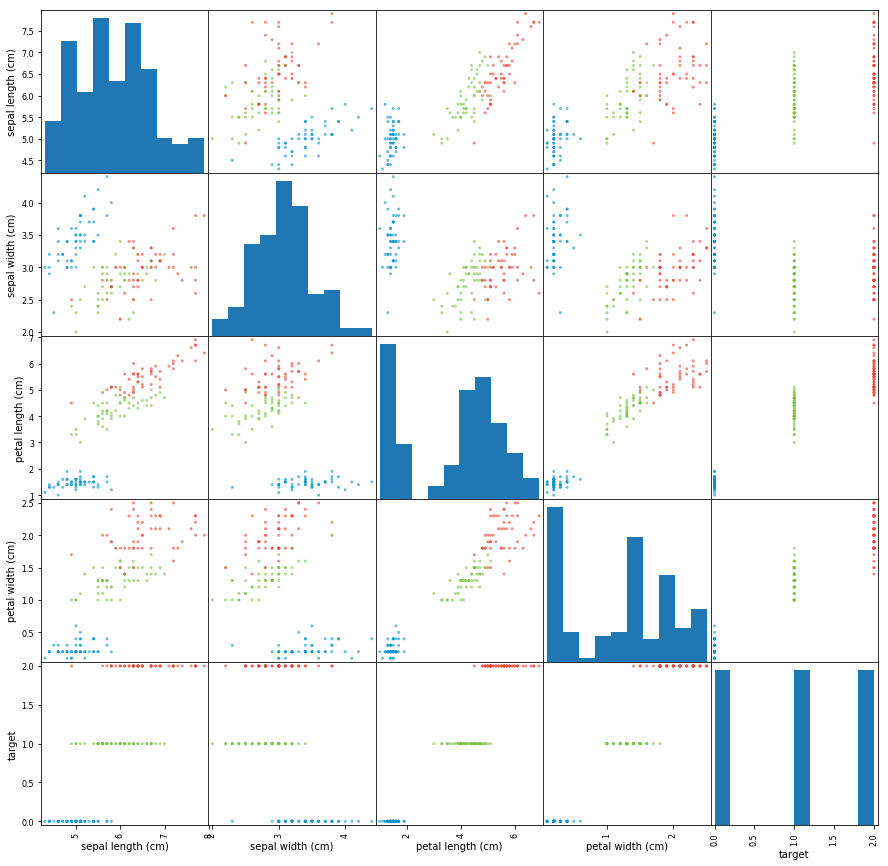We'll only select the first two flower classes for binary classification (~100 rows), and only use the first 2 features:

In :
# Select first 2 flower classes (~100 rows)
# And first 2 features

sepal_len = iris['data'][:100,0]
sepal_wid = iris['data'][:100,1]
labels = iris['target'][:100]


We will also center the data. In this case, removing the mean means that we won't need a bias in our model and still get reasonable results. Our binary classification model will look like this:

\begin{align*} z &= w_1 x_1 + w_2 x_2 \\ y &= \sigma(z) \end{align*}

If $y >= 0.5$ then we will classify the flower as a Setosa.

In :
sepal_len -= np.mean(sepal_len)
sepal_wid -= np.mean(sepal_wid)


Let's look at these two features. Note that in our case, the data set is linearly separable, meaning that it is possible to draw a line that separates the two classes.

In :
plt.scatter(sepal_len,
sepal_wid,
c=labels,
cmap=plt.cm.Paired)
plt.xlabel("sepal length")
plt.ylabel("sepal width")

Out:
Text(0, 0.5, 'sepal width')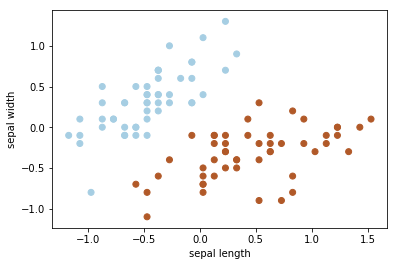## Decision Boundaries¶

We can show that $y = \sigma(z) >= 0.5$ if and only if $z >= 0$. Meaning that the decision boundary $y = 0.5$ can be expressed as $w_1 x_1 + w_2 x_2 = 0$. The decision boundary is therefore a line through the origin in the data space!

The following function will help us plot a decision boundary $w_1 x_1 + w_2 x_2 = 0$. (You're not required to know how this code works.)

In :
def plot_sep(w1, w2, color='green'):
'''
Plot decision boundary hypothesis
w1 * sepal_len + w2 * sepal_wid = 0
in input space, highlighting the hyperplane
'''
plt.scatter(sepal_len,
sepal_wid,
c=labels,
cmap=plt.cm.Paired)
plt.title("Separation in Input Space")
plt.ylim([-1.5,1.5])
plt.xlim([-1.5,2])
plt.xlabel("sepal length")
plt.ylabel("sepal width")
if w2 != 0:
m = -w1/w2
t = 1 if w2 > 0 else -1
plt.plot(
[-1.5,2.0],
[-1.5*m, 2.0*m],
'-y',
color=color)
plt.fill_between(
[-1.5, 2.0],
[m*-1.5, m*2.0],
[t*1.5, t*1.5],
alpha=0.2,
color=color)
if w2 == 0: # decision boundary is vertical
t = 1 if w1 > 0 else -1
plt.plot([0, 0],
[-1.5, 2.0],
'-y',
color=color)
plt.fill_between(
[0, 2.0*t],
[-1.5, -2.0],
[1.5, 2],
alpha=0.2,
color=color)


Let's look at a few example hypothesis to see how the choices of $w_1$ and $w_2$ influence the decision boundary:

In :
# Example hypothesis
#   sepal_wid = 0

plot_sep(0, 1)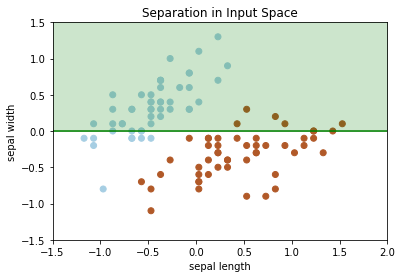In :
# Another example hypothesis:
#   -0.5*sepal_len + 1*sepal_wid >= 0

plot_sep(-0.5, 1)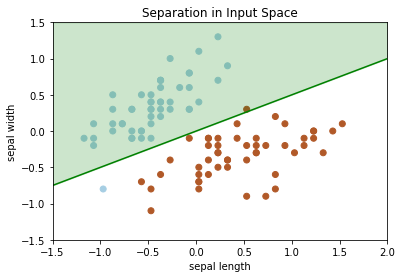In :
# Another example hypothesis:
#   -1.5*sepal_len + 3*sepal_wid >= 0

plot_sep(-1.5, 3)The decision boundary of the last two hypotheses look identical! Note that there is a difference between the two models. For a flower with (mean-adjusted) sepal_length = 0 and sepal_width = -0.5, the predictions for the two models are:

In :
z1 = -0.5 * 0 + 1 * (-0.5)
y1 = 1 / (1 + np.exp(-z1))
print("Prediction for model 2: ", y1)

z2 = -1.5 * 0 + 3 * (-0.5)
y2 = 1 / (1 + np.exp(-z2))
print("Prediction for model 2: ", y2)

Prediction for model 2:  0.3775406687981454
Prediction for model 2:  0.18242552380635635


The second model will be more "certain" about its predictions.

## Using sklearn¶

In project 1, you'll be writing code to use gradient descent to solve a linear classification problem. In practice, we use code that is already written and tested for us.

In :
import sklearn.linear_model
model = sklearn.linear_model.LogisticRegression(fit_intercept=False)
model.fit(np.stack([sepal_len, sepal_wid], axis=1),
labels)

/Users/xuexue/miniconda3/lib/python3.6/site-packages/sklearn/linear_model/logistic.py:432: FutureWarning: Default solver will be changed to 'lbfgs' in 0.22. Specify a solver to silence this warning.
FutureWarning)

Out:
LogisticRegression(C=1.0, class_weight=None, dual=False, fit_intercept=False,
intercept_scaling=1, max_iter=100, multi_class='warn',
n_jobs=None, penalty='l2', random_state=None, solver='warn',
tol=0.0001, verbose=0, warm_start=False)

Here are the coefficients that we get from sklearn:

In :
model.coef_

Out:
array([[ 3.02235857, -3.04217535]])
In :
plot_sep(3.02235857, -3.04217535)